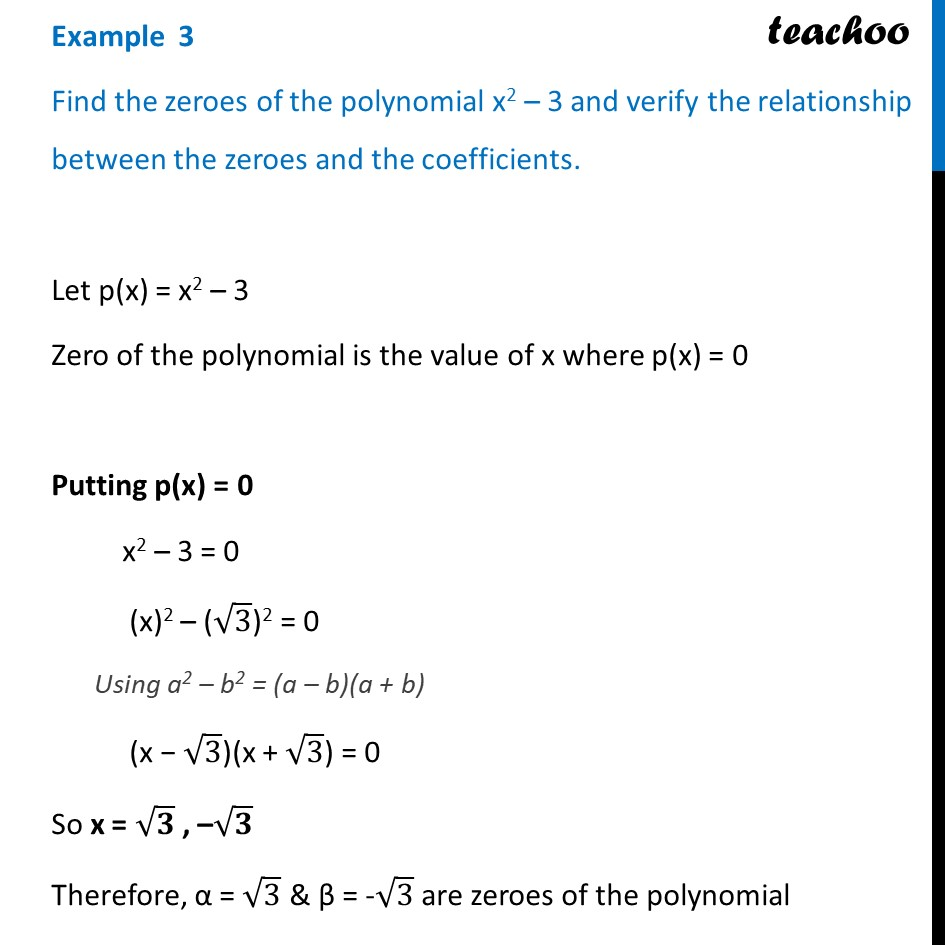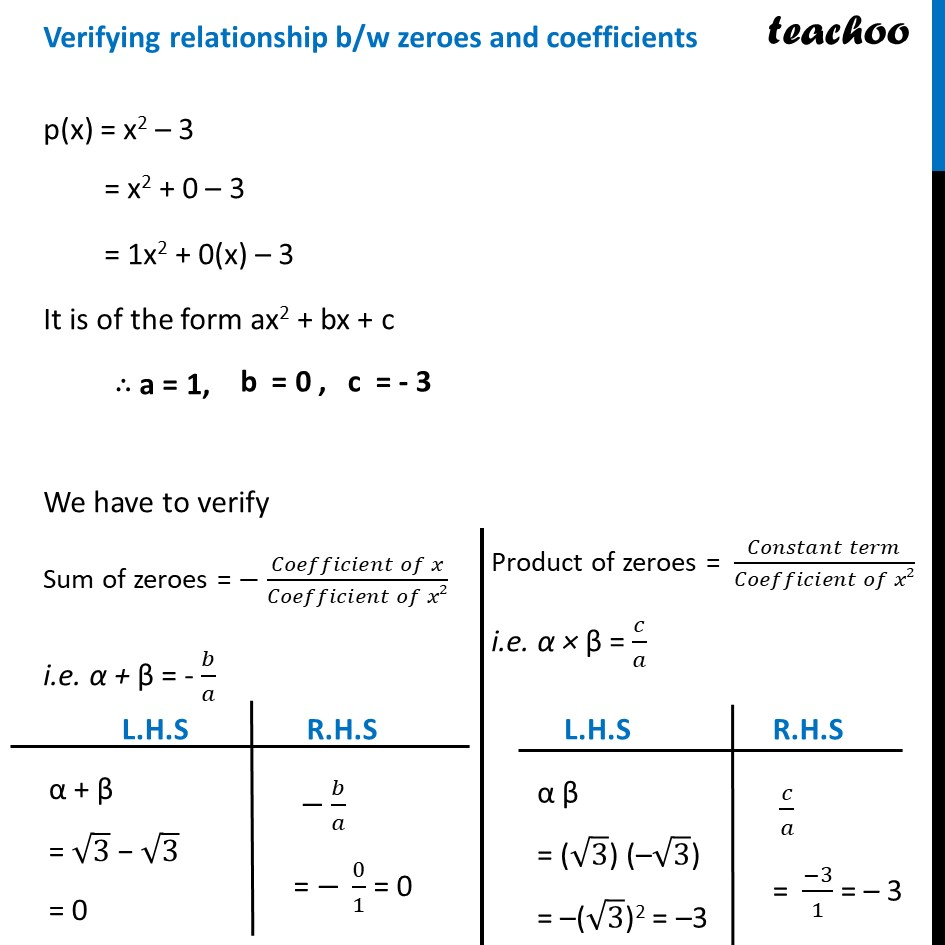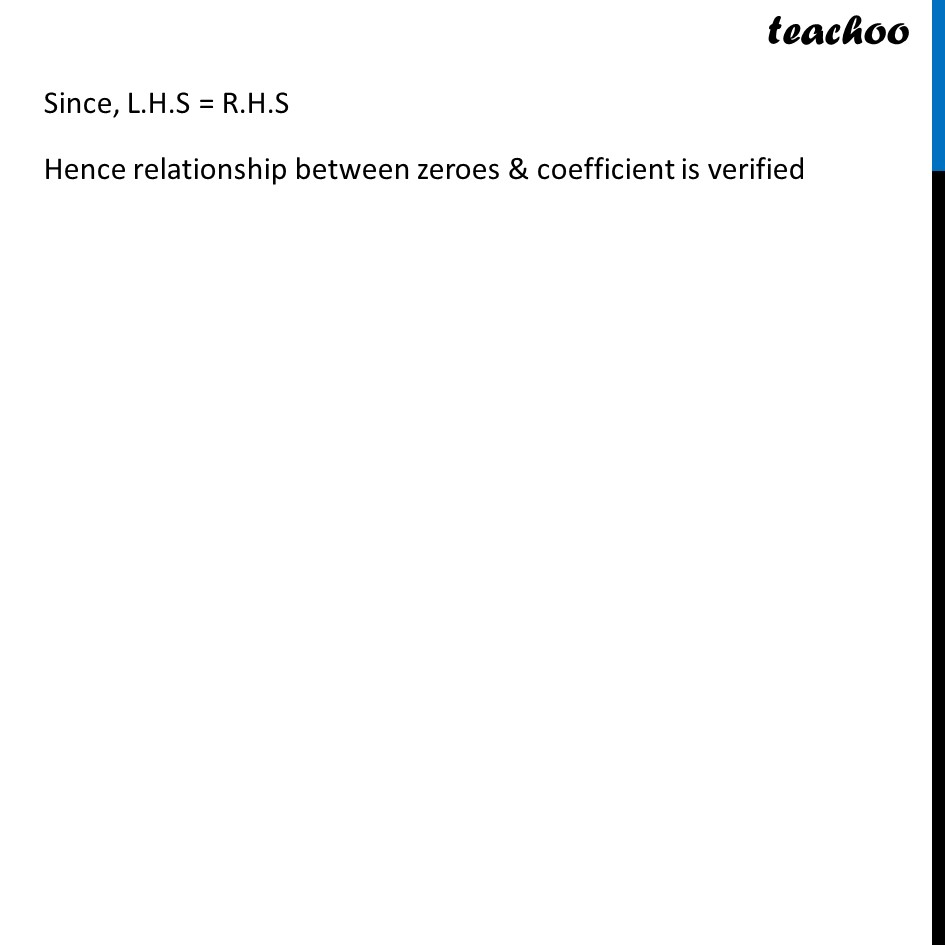Chapter 2 Class 10 Polynomials

Class 10
Important Questions for Exam - Class 10Learn in your speed, with individual attention - Teachoo Maths 1-on-1 Class

### Transcript

Example 3 Find the zeroes of the polynomial x2 – 3 and verify the relationship between the zeroes and the coefficients. Let p(x) = x2 – 3 Zero of the polynomial is the value of x where p(x) = 0 Putting p(x) = 0 x2 – 3 = 0 (x)2 – (√3)2 = 0 Using a2 – b2 = (a – b)(a + b) (x − √3)(x + √3) = 0 So x = √𝟑 , –√𝟑 Therefore, α = √3 & β = -√3 are zeroes of the polynomial Verifying relationship b/w zeroes and coefficients p(x) = x2 – 3 = x2 + 0 – 3 = 1x2 + 0(x) – 3 It is of the form ax2 + bx + c ∴ a = 1, We have to verify Sum of zeroes = − (𝐶𝑜𝑒𝑓𝑓𝑖𝑐𝑖𝑒𝑛𝑡 𝑜𝑓 𝑥)/(𝐶𝑜𝑒𝑓𝑓𝑖𝑐𝑖𝑒𝑛𝑡 𝑜𝑓 𝑥2) i.e. α + β = - 𝑏/𝑎 α + β = √3 − √3 = 0 − 𝑏/𝑎 = − 0/1 = 0 α β = (√3) (–√3) = –(√3)2 = –3 𝑐/𝑎 = (−3)/1 = – 3 Since, L.H.S = R.H.S Hence relationship between zeroes & coefficient is verified